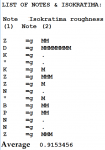Unusual cadence in the 3rd mode

Γεώργιος Καραγιαννάκης

Νεοσύλλεκτος
That's an explanation.

Attachments

• 139.8 KB Views: 28
• 162.5 KB Views: 30

Pappous43

Παλαιό Μέλος
This is an interesting case of ison.
We can apply the Helmhotz's method of evaluating the (average) best ison
i.e. the one having the smallest "Roughness" (Rauhigkeit in German).
The conclusion is that the best (single) ison for the phrase is ga.
Method:
1) Execute program BZQ.exe and copy+paste the following part of the score:
7s =g -Z -D K /" K |..Z Z | N " -<VB -P -N \N ..Z
=g means ison is g (ga)
2) Press small button i , to display information.
This is a part of the table, showing the ison used and the corresponding Roughness.3) The worst individual roughness is with the 2nd note Di which is about 8 (8 Ms).
Thus average roughness of ga ison is about 0.9
If we try other isons (or "isa") we get the following results:
Ison Roughness
=n 3.8
=p 2.4
=b 6.8 worst
=g 0.9 best
=D 1.1
=K 6.2
=Z 2.0

If we want to make a small refinement to improve the effect, we can use D ison on the 2nd note (D).
The score in bzq notation will be:
7s =g -Z =D-D =g K /" K |..Z Z | N " -<VB -P -N \N ..Z
The result is an average Roughness of 0.6.
Here is the sound result generated by BZQ, using a virtual choir: